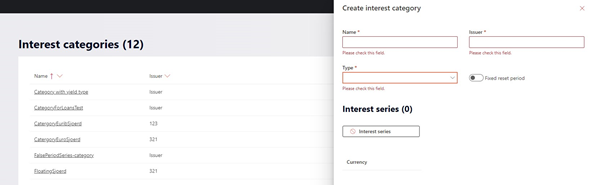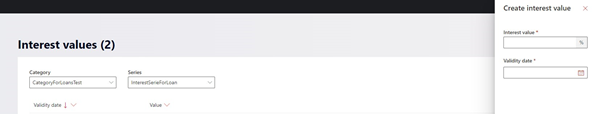# Introduction

Ability to define variable (floating) interest rates, which can be used on loans. For example: the floating interest rate or the floating penalty rate both set on the loan template.

# Interest management

Interest category \ series
Ability to create an interest category with a series.

Creating a new interest category: ‘Interest categories’ > ‘Create interest category’Name
The name of the interest category

Issuer
A free format description of the issuer of the interest category

Type
The type of the interest category, the following types are supported:

• Interest, used to define the base interest rate. Can be either fixed interest period or average interest.
• Interest spread, the spread on top of the base interest.
• Penalty rate, the penalty interest (rate) can be defined as a floating rate coming from a specific penalty interest (rate) category, applied when repayment is late. Can be selected on the template.
• Yield, this type of category is used to calculate when fair value is used to calculate pay up fee. The pay up fee settings table will reference the fair value category to be used. This category should represent the market value rating for the type of loan being calculated.

Interest series
Ability to define the currency related to the category, could have multiple unique series (so with unique currencies) within a category.

Slider – fixed reset period
Ability to define if a category has a fixed interest period where a new rate should be applied, in x year\months\weeks\overnight (currently only months are supported)
Fixed interest period cannot be overlapping if they have the same currency and period. When set, the loan created with this characteristic will get the interest value at the beginning of the period (for the set period)."

Interest Values
Ability to define the interest category\series values, the rates.

Creating a new values: ‘Interest values’ > ‘Create interest value’Interest value
The interest value percentage, cannot be negative?

Validity date
The date from which the entered rate value will be applied, per date there can be only one value (rate).